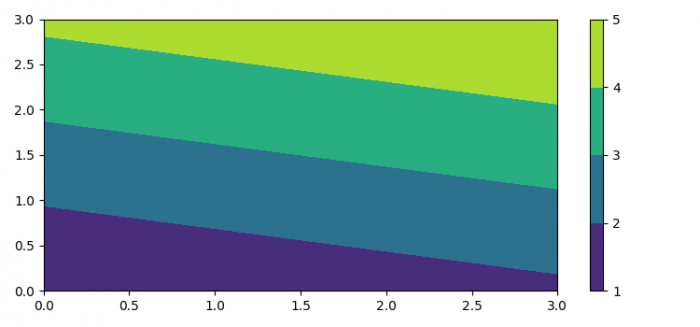# Matplotlib colorbar background and label placement

To have colorbar background and label placement, we can take the following steps −

• Set the figure size and adjust the padding between and around the subplots.
• Create random data using numpy.
• Plot the contours.
• With scalar mappable instance, make the colorbar.
• Set ticklabels for colorbar with background and label placement
• To display the figure, use show() method.

## Example

import numpy as np
import matplotlib.pyplot as plt

plt.rcParams["figure.figsize"] = [7.50, 3.50]
plt.rcParams["figure.autolayout"] = True

data = np.linspace(0, 10, num=16).reshape(4, 4)

cf = plt.contourf(data, levels=(0, 2.5, 5, 7.5, 10))
cb = plt.colorbar(cf)
cb.set_ticklabels([1, 2, 3, 4, 5])

plt.show()

## Output